# Elliptic partial differential equation, numerical methods

(diff) ← Older revision | Latest revision (diff) | Newer revision → (diff)

Methods for the approximate determination of solutions of elliptic partial differential equations. Among the various classes of problems that are raised for elliptic equations, boundary value problems and problems with Cauchy data have been most thoroughly studied. The latter are ill-posed and require for their solution special methods . More typical for elliptic equations are boundary value problems, and for their approximate solution many different numerical methods have been worked out (see , ). In computational practice grid methods are the most widespread, and among them the method of finite differences (see Difference methods; Difference schemes, theory of, , ) and the method of finite elements (see ). Although these methods differ in their approach to the construction of an approximate solution — in the first one approximates the equation and the boundary conditions (see Approximation of a differential boundary value problem by difference boundary value problems) and in the second one approximates the required solution itself — nevertheless the resulting algebraic systems for determining an approximate solution are often based on similar ideas and in a number of cases coincide completely.

The essence of the method of finite differences consists of the following. The domain of continuous variation of the arguments of the original problem is replaced by a discrete set of points (nodes), which is called a grid; derivatives occurring in the differential equation and the boundary conditions are approximated by difference relations; here boundary value problems for the differential equations are replaced by a system of algebraic equations (a difference scheme). If the resulting difference boundary value problem is solvable (possibly on a sufficiently fine grid) and if its solution for a sufficiently refined grid approximates (converges to) a solution of the original problem for the differential equation, then a solution of the difference problem obtained on any fixed grid is accepted as an approximate solution of the original problem.

The simplest example of an elliptic partial differential equation is the Poisson equation (the Laplace equation when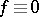):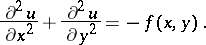(1)

Examples of difference schemes for the Poisson equation are given in the articles Boundary value problem, numerical methods for partial differential equations and Difference equation.

In a finite element method a generalized solution of a boundary value problem is approximated. If, for example, (1) is given for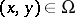and one examines for it the homogeneous Dirichlet problem, that is,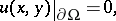(2)

whereis the boundary of, then a generalized solution of (1)–(2) is defined as a function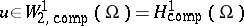that for any function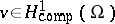satisfies the integral identity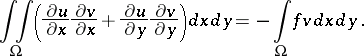(3)

Hereis the Sobolev space of functions that vanish on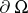(as generalized functions). The most important finite element methods are those of Galerkin type (conformal finite element methods). In the Galerkin method an approximate solution is sought in a finite-dimensional subspace of the space on which the integral identity that defines a generalized solution is given. Applied to the problem (3) a Galerkin-approximate solution is a functionthat for any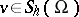satisfies the integral identity (3). In a finite element method the subspace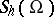must have certain special properties (see Difference scheme, variational).

The specific nature of the finite-dimensional subspace that distinguishes the finite element method from other realizations of the Galerkin method is illustrated by the following examples. Suppose that the domainin which a solution of (1)–(2) is sought is a polygon. Thenis partitioned into small triangles (the finite elements) so that any two do not have common points at all, or just one common vertex, or one common side. As a finite-dimensional subspace of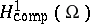one takes the spaceof functions that are piecewise linear, linear on every triangle, continuous, and vanish on. The dimension ofis the number of vertices of the triangle (without multiplicities) that do not hit the boundary. These vertices are called nodes. As a basis ofone can take the collection of elementsthat differ from zero only at one node. A characteristic feature of this basis is the fact that for every element its support is minimal and is formed by the union of all triangles with a common vertex at that node where the given basis element is non-zero. Thanks to this property, on every finite element there are at most three non-zero basis functions (among the vertices that do not hit). This allows one to use the restrictions of these basis functions to finite elements as a basis on the finite elements and to perform all calculations on finite elements without bringing in information on other finite elements. This property makes the finite element method very effective from the point of view of its implementation .

If, for example,is the unit square and the partition ofinto finite elements is effected by the three families of equidistant lines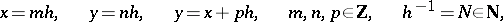under the condition that the non-zero values of the basis functions are 1, then for the coefficientsof the expansion of the approximate solutionone obtains a system of equations that agrees entirely with the system obtained from the finite difference method. Here theare the values of the approximate solution at the nodes.

The accuracy of the finite element method thus described is characterized by the estimatewhereis the largest linear dimension of the finite elements. To achieve higher accuracy one has to look for an approximate solution not in the space of piecewise-linear functions but in that of piecewise-quadratic or, more generally, piecewise-polynomial functions. In this case the accuracy for a suitable smoothness of the required solution is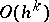, whereis the degree of the polynomials used.

Apart from triangular finite elements one also uses quadrilateral finite elements. However, when the sides of the quadrilaterals are not parallel to the coordinate axes, one has to employ the isoparametric technique, that is, begin by mapping the finite elements in question into canonical form (in the present case into rectangles with sides parallel to the coordinate axes) by means of a non-degenerate transformation whose inverse is given by the same functions as the approximate solution on canonical finite elements. One can use triangle and quadrilaterals with curvilinear sides (again applying the isoparametric technique), which is necessary to solve problems in domains with smooth boundaries by methods of higher order of accuracy than the first.

Apart from the finite element methods of Galerkin type there are also other so-called non-conformal finite element methods, in which the solution is sought in spaces other than subspaces of the original ones. Most frequently such methods are used for problems with elliptic partial differential equations of an order higher than the second.

The methods of finite differences and of finite elements lead to systems of linear algebraic equations of large order with sparse matrices; one can suppress the majority of the zero elements of these matrices (see , ). Yet another method of approximate solution of boundary value problems for elliptic partial differential equations has been significantly developed: the method of boundary elements .

How to Cite This Entry:
Elliptic partial differential equation, numerical methods. Encyclopedia of Mathematics. URL: http://encyclopediaofmath.org/index.php?title=Elliptic_partial_differential_equation,_numerical_methods&oldid=11982
This article was adapted from an original article by V.B. Andreev (originator), which appeared in Encyclopedia of Mathematics - ISBN 1402006098. See original article